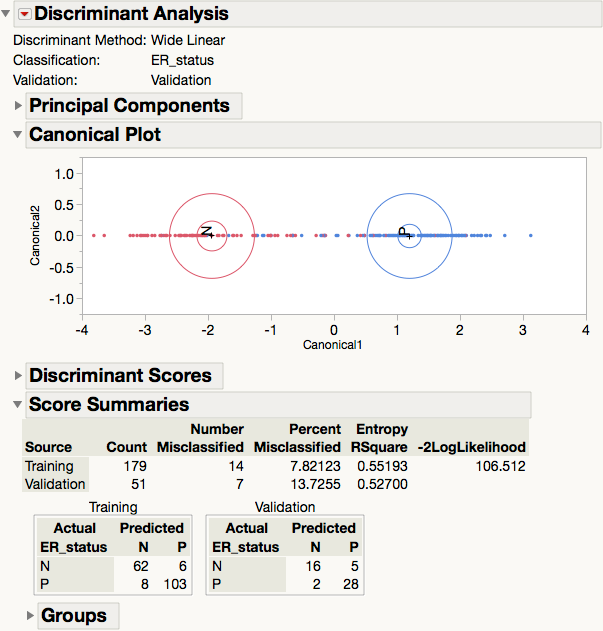Choose Language Hide Translation Bar
Wide data discriminant analysis

With multivariate methods, you have to do things differently when you have wide (many thousands of columns) data.

The Achilles heel for the traditional approaches for wide data is that they start with a covariance matrix, which grows with the square of the number of columns. Genomics research, for example, tends to have wide data:

• In one genomics study, there are 10,787 gene expression columns, though there are only 230 rows. That covariance matrix has more than 58 million elements and takes 465MB of memory.
• Another study we work with has 49,041 genes on 249 individuals, which results in a covariance matrix that uses 9.6GB of memory.
• Yet another study has 1,824,184 gene columns, yielding a covariance matrix of 13.3TB. Computers just don’t have that much memory – in fact, they don’t have that much disk space.
• Even if you have the memory for these wide problems, it would take a very long time to calculate the covariance matrix, on the order of n*p2, where n is the number of rows, and p is the number of columns. Furthermore, it gets even worse when you have to invert the covariance matrix, which would cost on the order of p3. In addition, the covariance matrix is very singular, since there are far fewer rows than columns in the data.

So you would think that multivariate methods are impractical or impossible for these situations. Fortunately, there is another way, one that makes wide problems not only practical, but reasonably fast while yielding the same answers that you would get with a huge covariance matrix.

The number of effective dimensions in the multivariate space is on the order of the number of rows, since that is far smaller than the number of columns. All the variation in that wide matrix can be represented by the principal components, those associated with positive variance, i.e., non-zero eigenvalues. There is another way to get the principal components using the singular value decomposition of the (standardized) data. Now that you are in principal component space, the dimension of these principal components is small; in fact, all the calculations simplify because the covariance matrix of principal components is diagonal, which is trivial to invert.

For example, let’s look at that genomics data set with 1,824,184 columns across 249 rows. I was able to do the principal components of that data using JMP 12 on my 6-core Mac Pro in only 94 minutes. Doing it the other way would clearly have been impossible.

With discriminant analysis, the main task is to calculate the distance from each point to the (multivariate) mean of each group. However, that distance is not the simple Euclidean distance, but a multivariate form of the distance, one called the Mahalanobis distance, which uses the inverse covariance matrix.

But think about the standardized data – the principal components are the same data, but rotated through various angles. The difference is that the original data may have 10,000 columns, but the principal components only have a few hundred, in the wide data case. So if we want to measure distances between points or between a point and a multivariate mean (centroid), then we can do it in far fewer dimensions. Not only that, but the principal coordinates are uncorrelated, which means that the matrix in the middle of the Mahalanobis distance calculations is diagonal, so the distance is just a sum of squares of individual coordinate distances.

We take the principal components of the mean-centered data by group. Then we add back the mean to this new reduced-dimension data. The transformed mean is just the principal components transformation of the original group means.

### Example

There is a set of breast cancer genome expression data that is a benchmark used by the Microarray Quality Consortium with 230 rows and 10,787 gene expressions. A discriminant analysis using the wide linear method takes only 5.6 seconds (on Mac Pro) and produces a cross-validation misclassification rate of only 13.7% on the hardest-to-classify category, ER_status. This misclassification rate is close to the best achieved for this problem, given that the other methods are expensive.Note: This is part of a Big Statistics series of blog posts by John Sall. Read all of his Big Statistics posts.

Article Labels

There are no labels assigned to this post.

Article Tags